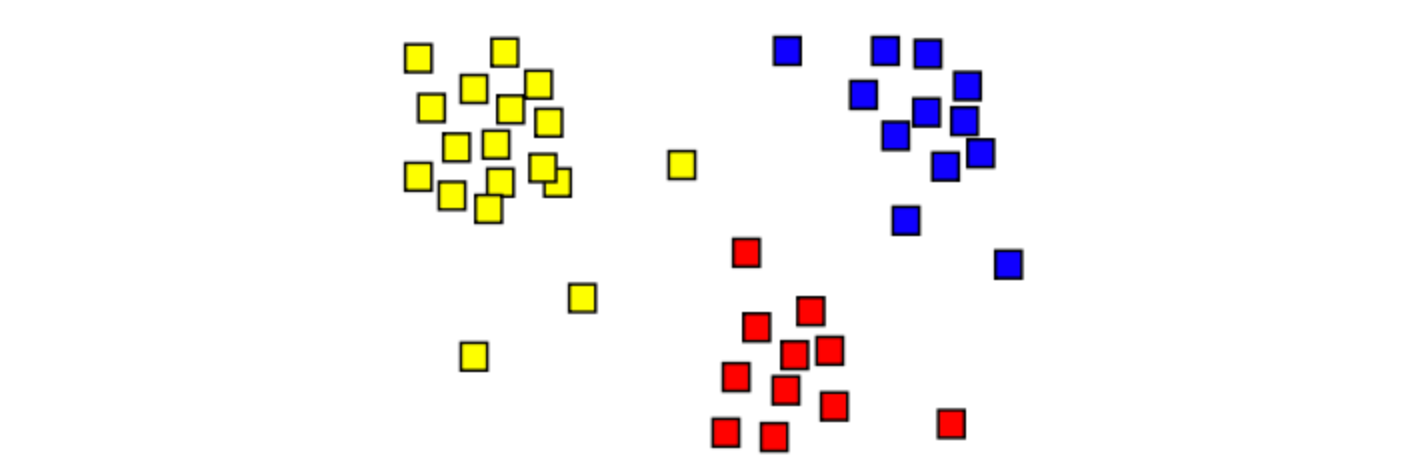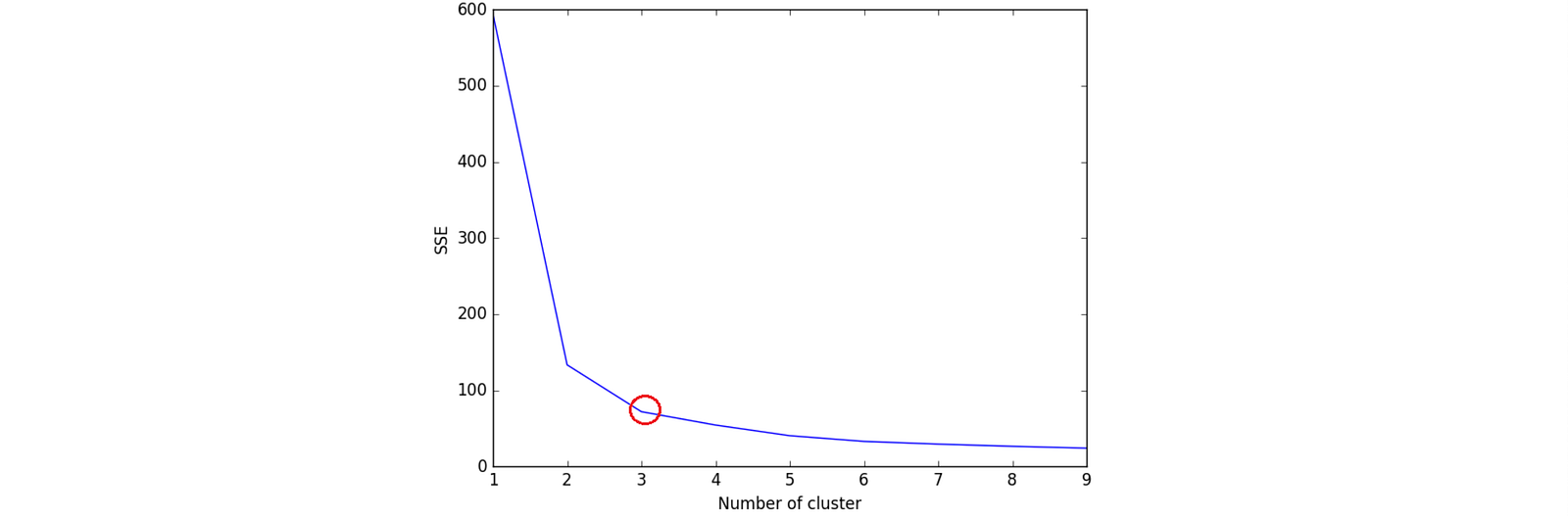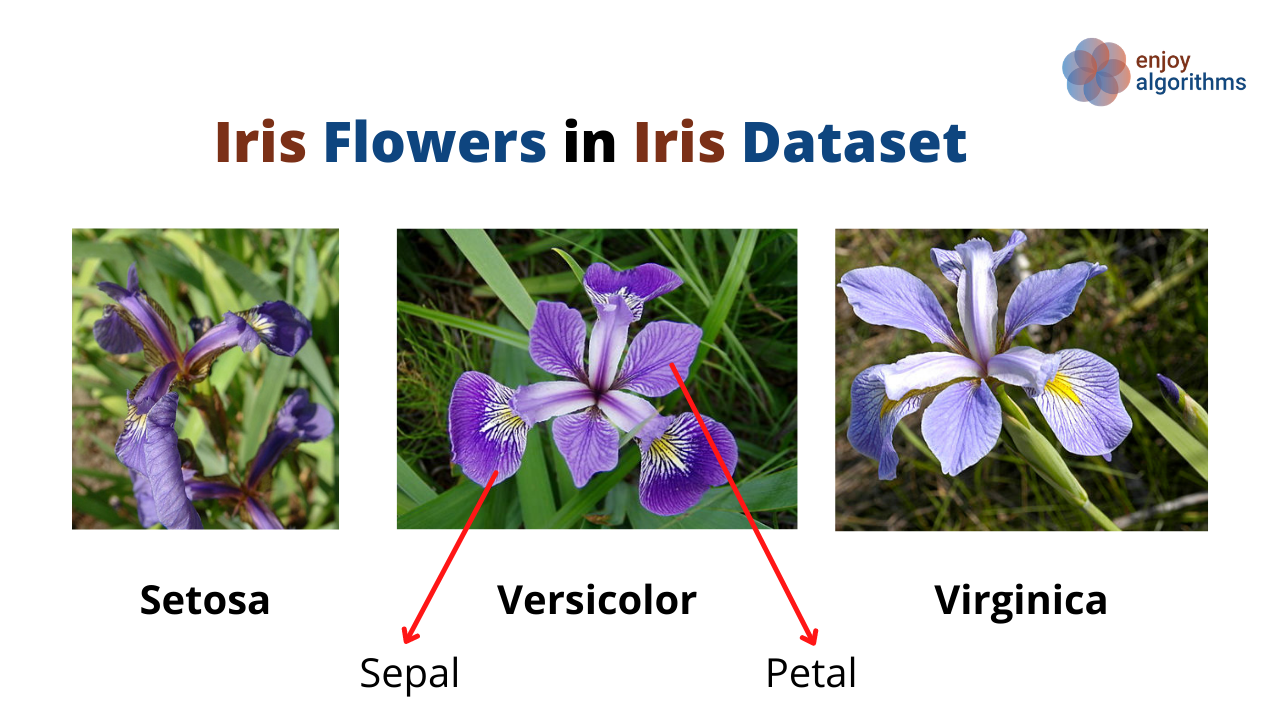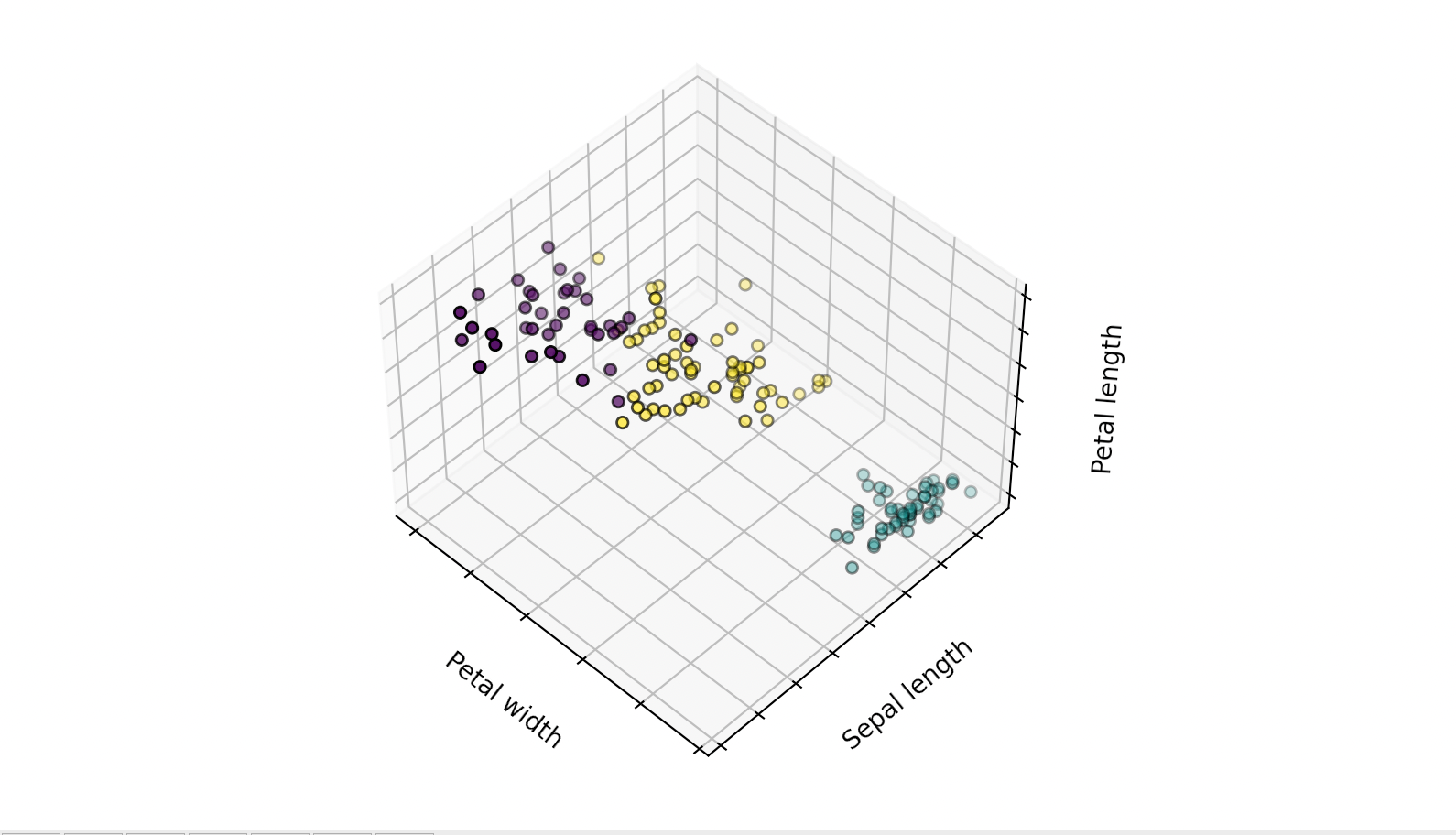# K-Means Clustering Algorithm In Machine Learning

Data understanding is very crucial in the field of Machine Learning. With the recent trend of collecting data, every organization has started collecting some form of data, but the concern is, most of these data are unutilized and considered digital junk. Annotating or Labeling this vast amount of data with the help of annotation engineers would be time-consuming.

In such a scenario, unsupervised learning algorithms come into existence that can extract meaningful information from the junk and present it in a human-readable format. Clustering is one of them.

### Key takeaways from this blog would be:

1. What is clustering?
2. What are the K-Means algorithm and its pseudocode?
3. How to choose the value of K in K-Means?
4. What are the possible improvements in K-Means?
5. What are some inherent problems with clustering algorithms?

K-means is a type of clustering algorithm; hence, before moving towards it, let’s formally define the term “Clustering.”

Clustering is an unsupervised learning technique which is used to find the subgroups (also known as Clusters) in the dataset.

Data samples have been divided into three clusters in the image below, and each cluster has been assigned different colors.Clusters

It is popular in many fields, and hence there exist many algorithms to perform this task of clustering. K-means is one of them. The term “k-means” was first used by James MacQueen in 1967. It is an older technique but still very popular in the data science and machine learning industries. If we define the term formally,

K-means is a simple and elegant approach which is used to partition data samples into a pre-defined K distinct and non-overlapping clusters.

The value of K in the k-means algorithm depends upon the choice of the user. In the image above, the user has defined the value of K = 3. Every observation from the dataset will follow two fundamental properties,

1. Each observation belongs to at least of the K clusters.
2. No observation will belong to more than one cluster.

Because of its simplicity, relative robustness (work with a broader variety of datasets), and “good enough” explanation, it is still considered one of the first algorithms that data analysts use to investigate a new dataset.

### Understanding of Algorithms in a Step-wise manner

Let’s suppose we have some data samples (X1, X2, …, Xn), and we want to divide these data samples into K-different clusters. K-means work with the iterative logic, and that can be summarized in the following five steps,

Step 1: Accept inputs of data and the number of clusters to group that data. There are n data samples in our case, and we want to divide these samples into k different clusters.

Step 2: Initialize the first K clusters.

• Pick first k samples, or
• Take Random sampling of k elements from the available dataset.

These k samples will be treated as temporary centroids (also called mean).

Step 3: Now we have (n-k) data samples left. Based on the proximity to the k centroid values, each data sample from (n-k) will be assigned to only one of the k clusters. At this stage, all the samples have been assigned to some cluster.

• Each record is assigned to the nearest cluster using a measure of distance (e.g., Euclidean distance)

Step 4: A new centroid is calculated by calculating the arithmetic means of data samples present in each cluster for each cluster. At this step, we will have k centroids which can be different from the earlier chosen k samples.

Step 5: Iterating over the same algorithm starting from stage 3 to the entire n samples. This will be repeated until we see no/negligible movement of samples among clusters.

Via this way, it groups the data samples into the desired number of clusters. Yet this is a vital algorithm, but it has certain limitations as well.

### Limitations of K-Means

• This algorithm is computationally expensive and requires time proportional to the product of the number of data items (n), number of clusters (k), and the number of iterations (m).
• It generally converges to a local optimum because the quality of the resulting clusters highly depends upon the selection of initial k clusters.
• It can suffer to an empty cluster problem.

### Possible Improvements

As per the limitations discussed above, there are many scopes where this algorithm can be improved from its native version. If we have noticed, in each iteration, the k-means algorithm computes the distance between data samples and the centroid within each cluster. It is making this algorithm computationally very expansive in the case of huge datasets. However, we can utilize the knowledge of the distance calculated in the previous iteration to make it computationally cheaper. Can you think how?

In the first iteration, we calculated the distance for each data point to the nearest cluster and suppose we termed it as prev_distance[i] for ith sample. At the next iteration, we will calculate the distance of the ith sample to the previous nearest cluster ( but with the updated centroid) and compare it with the previous distance prev_distance[i]. There will be no movement of that sample within clusters if the new distance is less than or equal to the previous distance. By this, we will be saving the time required to compute the distances to k-1 clusters.

### How to decide the number of clusters k in the K-Means algorithm?

The decision of an optimal number of clusters is subjective and depends upon the methods to find the similarity based on which we performed the clustering. There are some famous methods like,

#### Elbow method

1. Run the k-means algorithm for different values of k, let’s say k=1 to k=10.
2. For every k, calculate the total sum of distances of samples to their closest cluster centroid. Let’s say it SSE.
3. Plot the curve of SSE with respect to the number of clusters k.
4. The value of k at the bend (knee) in the plot is generally considered an indicator of the appropriate number of clusters.
``````SSE = {}
for k in range(1, 10):
k_means = KMeans(n_clusters=k, max_iter=1000).fit(data)
data["clusters"] = kmeans.labels_
SSE[k] = kmeans.inertia_
# Inertia: Sum of distances of samples to their closest cluster #center
plt.figure()
plt.plot(list(SSE.keys()), list(SSE.values()))
plt.xlabel("Number of cluster")
plt.ylabel("SSE")
plt.show()
``````We can say that the optimal value of k is 3 for the data used in the above code.

#### Average silhouette method

1. Run the k-means algorithm for different values of k, let’s say k=2 to k=11.
2. For every k, calculate the average silhouette of observations. Let’s say AvS.
3. Plot the curve of AvS with respect to the number of clusters k.
4. The value of k at the maximum AvS will be considered optimal.
``````for n_cluster in range(2, 11):
kmeans = KMeans(n_clusters=n_cluster).fit(data)
label = kmeans.labels_
AvS = silhouette_score(data, label, metric='euclidean')
print("For n_clusters={}, The Silhouette Coefficient is {}".format(n_cluster, AvS))
For n_clusters=2, The Silhouette Coefficient is 0.680813620271
For n_clusters=3, The Silhouette Coefficient is 0.552591944521
For n_clusters=4, The Silhouette Coefficient is 0.496992849949
For n_clusters=5, The Silhouette Coefficient is 0.488517550854
For n_clusters=6, The Silhouette Coefficient is 0.370380309351
For n_clusters=7, The Silhouette Coefficient is 0.356303270516
For n_clusters=8, The Silhouette Coefficient is 0.365164535737
For n_clusters=9, The Silhouette Coefficient is 0.346583642095
For n_clusters=10, The Silhouette Coefficient is 0.328266088778
``````

Here, n = 2 has the highest coefficient, so ideally, it should be selected, but, based on the knowledge of data, we were aware that 3 species are there, and hence the next value of 3 is selected for optimal. When dealing with higher dimensions, this method produces a better result.

#### Implementation

Let’s try to implement this algorithm using the Scikit-Learn library on one of the famous datasets of the framework, i.e., the Iris Dataset shown in the image below.Step 1: Importing the required libraries of pandas, Iris datasets, KMeans model, and matplotlib.

``````import pandas as pd
from sklearn.cluster import KMeans
import matplotlib.pyplot as plt
from mpl_toolkits.mplot3d import Axes3D
``````

Step 2: Extract the data from the load_iris dataset and separate data and target variables.

``````iris = load_iris()
X = iris.data
y = iris.target
``````

Step 3: Fit the Scikit-learn KMeans model.

``````kmeans = KMeans(n_clusters=3, max_iter=1000).fit(X)
labels = kmeans.labels_
``````

Step 4: Plot the scattered pred

``````plt.figure(figsize=(4, 3))
ax = Axes3D(fig, rect=[0, 0, .95, 1], elev=48, azim=134)
ax.scatter(X[:, 3], X[:, 0], X[:, 2],
c=labels.astype(float), edgecolor='k')
ax.w_xaxis.set_ticklabels([])
ax.w_yaxis.set_ticklabels([])
ax.w_zaxis.set_ticklabels([])
ax.set_xlabel('Petal width')
ax.set_ylabel('Sepal length')
ax.set_zlabel('Petal length')
ax.dist = 12
plt.show()
``````We can see that the 3 clusters are formed based on the Petal width, Petal Length, and Sepal Length.

### Practical Issues in Clustering

There is no doubt that clustering is one of the most important processes for data science and machine learning, but it has some practical challenges for which the solution is difficult to find. Some of these practical issues are

1. Performing normalization or standardization before applying cluster sometimes produce better results and sometimes worse. So this decision is not firm and only be observed by actually implementing it. And the final clusters are highly dependent upon these steps.
2. Choice of the number of clusters is not fixed and only can be observed by actually implementing it.
3. The results of the clustering algorithms are hard to analyze in the case of higher dimensions. Methods like PCA can be used to reduce the dimensions, but it causes data loss as well.

### Possible Interview Questions

k-means is considered one of the fundamental algorithms that every interviewer expects that candidate knows about it. Data analysis and finding patterns in the massive chunk of data is the most crucial step, and knowledge of k-means suggests that the data scientist position candidates have worked on unstructured data. Possible interview questions on this topic would be,

1. What is the step-wise process in which k-means find clusters?
2. How do we decide the number of clusters?
3. How can we improve the speed of the native k-means algorithm?
4. What are the inherent problems of clustering algorithms?
5. What are the other methods used for clustering?

### Conclusion

In this article, we discussed the k-means algorithm in a detailed fashion. We learned the pseudo-code of k-means algorithms, inherent problems associated with the k-Means algorithm, and how we can improve the time performance of the k-means algorithm. We discussed methods that can help find the optimal values k, one of the most frequent questions asked in the machine learning interviews. After that, we actually implemented the algorithm on the Iris dataset.

### Enjoy Learning! Enjoy Clustering! Enjoy Algorithms!

Subscribe to get well-designed content on data structures and algorithms, machine learning, system design, oops, and mathematics. enjoy learning!

### We Welcome Doubts and Feedback!